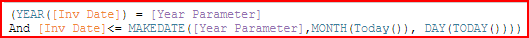# MAKEDATE

MAKEDATE is a useful function when you need to create a date value based on multiple information sources.
In the following examples the Year part of the date is provided by a Parameter field. The Month and Day parts of the date are provided by Today’s date using the Today function. This is often used for YTD & MTD filter/selection calculation fields.

### Year to Date (YTD) Calculated Filter as of Today’s month/day using a Year Parameter and the MAKEDATE function

This example uses the MAKEDATE function combining the year based on the user parameter with the month and day from the computer’s system date to create a YTD date filter.

1. Create an Integer parameter field to select the base year and show it so the user can enter a value.

2. Use the Parameter value with the MAKEDATE function to create a date based on the selected year and today’s Month & Day of the year.

3. Use the MAKEDATE value in the T|F calculation to evaluate when the Year of the Invoice Date equals the Year parameter value, and the Month/Day of the Invoice Date is less than or equal to today’s Month/Day.T|F calculation evaluating if the invoice date is <= this day in the selected parameter year

4. If you want to create a T|F calculation to evaluate YTD dates for multiple years, add to the existing logic adding or subtracting from the Year Parameter for the Year part of the date. In the example below, the OR command was added with another date condition for [Year Parameter] - 1

### Month to Date (MTD) Filter Calculation as of Today’s month/day using a Year Parameter and the MAKEDATE function

Similar logic to the YTD calculated filter can be used to make a MTD calculated filter.
In the YTD example, the year was selected based on the Parameter value. In the MTD example, add
(MONTH ([Inv Date] = MONTH (TODAY ()) to select the specific month. This needs to be added to both sections of the calculation for the current and prior year.

Note that the examples above do not consider Fiscal calendar differences.
DataSelf has created other T|F calculated fields that do the same function accounting for the Fiscal Calendar differences. If the fiscal calendar started on April 1st, The Inv Date Options, YTD Fiscal calculated field will account for this.
Fiscal calendars that don’t start at the beginning of a month require custom configuration of your DataSelf account.